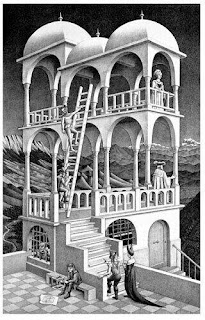Tuesday, November 06, 2007

What is a theory in physics?In terms of mathematical logic, a theory is a set of sentences, in some language, which is 'closed' under logical entailment. In other words, any sentence which is entailed by a subset of sentences from the theory, is itself already an element of the theory. Now, physicists mean something slightly different from this when they refer to something as a theory; a theory in physics is more akin to a class of 'models'.

In this context, a model for a set of sentences is an 'interpretation' of the langauge in which those sentences are expressed, which renders each sentence as true. In this context, an intepretation of a language identifies the domain over which the variables in the language range; it identifies the elements in the domain which correspond to the constants in the language; it identifies which elements in the domain possess the predicates in the language, which n-tuples of elements are related by the n-ary relations in the language, and which elements in the domain result from performing n-ary operations upon n-tuples in the domain.

Each theory in mathematical physics has a class of models associated with it. As Philosopher of Physics John Earman puts it, "a practitioner of mathematical physics is concerned with a certain mathematical structure and an associated set M of models with this structure. The...laws L of physics pick out a distinguished sub-class of models,...the models satisfying the laws L (or in more colorful, if
misleading, language, the models that 'obey' the laws L)."

The laws which define a class of mathematical models, therefore define a theory as far as physicists are concerned. If one retains the same general class of mathematical structure, but one changes the laws imposed upon it, then one obtains a different theory. Thus, for example, whilst general relativity represents space-time as a 4-dimensional Lorentzian manifold, if one changes the laws imposed by general relativity upon a Lorentzian manifold, (the Einstein field equations), then one obtains a different theory.

Physicists find that, at a classical level, the equations of a theory can be economically specified by something called a Lagrangian, hence physicists tend to identify a theory with its Lagrangian. In superstring theory, there are five candidate theories precisely because there are five candidate Lagrangians. This point is particularly crucial because it also explains why physicists associate different theories with different 'vacua'.

The Lagrangians of particle physics typically contain scalar fields, such as the Higgs field postulated to exist by the unified electroweak theory. These scalar fields appear in certain terms of the Lagrangian. The scalar fields have certain values which constitute minima of their respective potential energy functions, and such minima are called vacuum states (or ground states). If one assumes that in the current universe such scalar fields reside in a vacuum state (as the consequence of a process called symmetry breaking), then the form of the Lagrangian changes to specify this special case. After symmetry breaking, the Lagrangian is not the Lagrangian of the fundamental theory, but an 'effective' Lagrangian. Hence, the selection of a vacuum state changes the form of the Lagrangian, and because a Lagrangian defines a theory, the selection of a vacuum state for a scalar field is seen to define the selection of a theory. Physicists therefore tend to talk, interchangeably, about the number of possible vacua, and the number of possible theories in string theory.